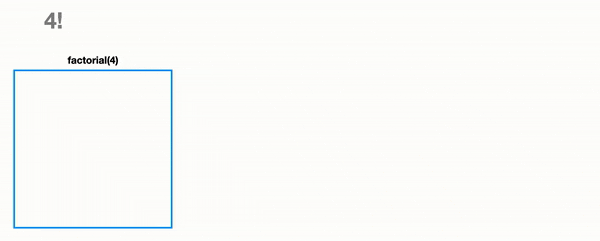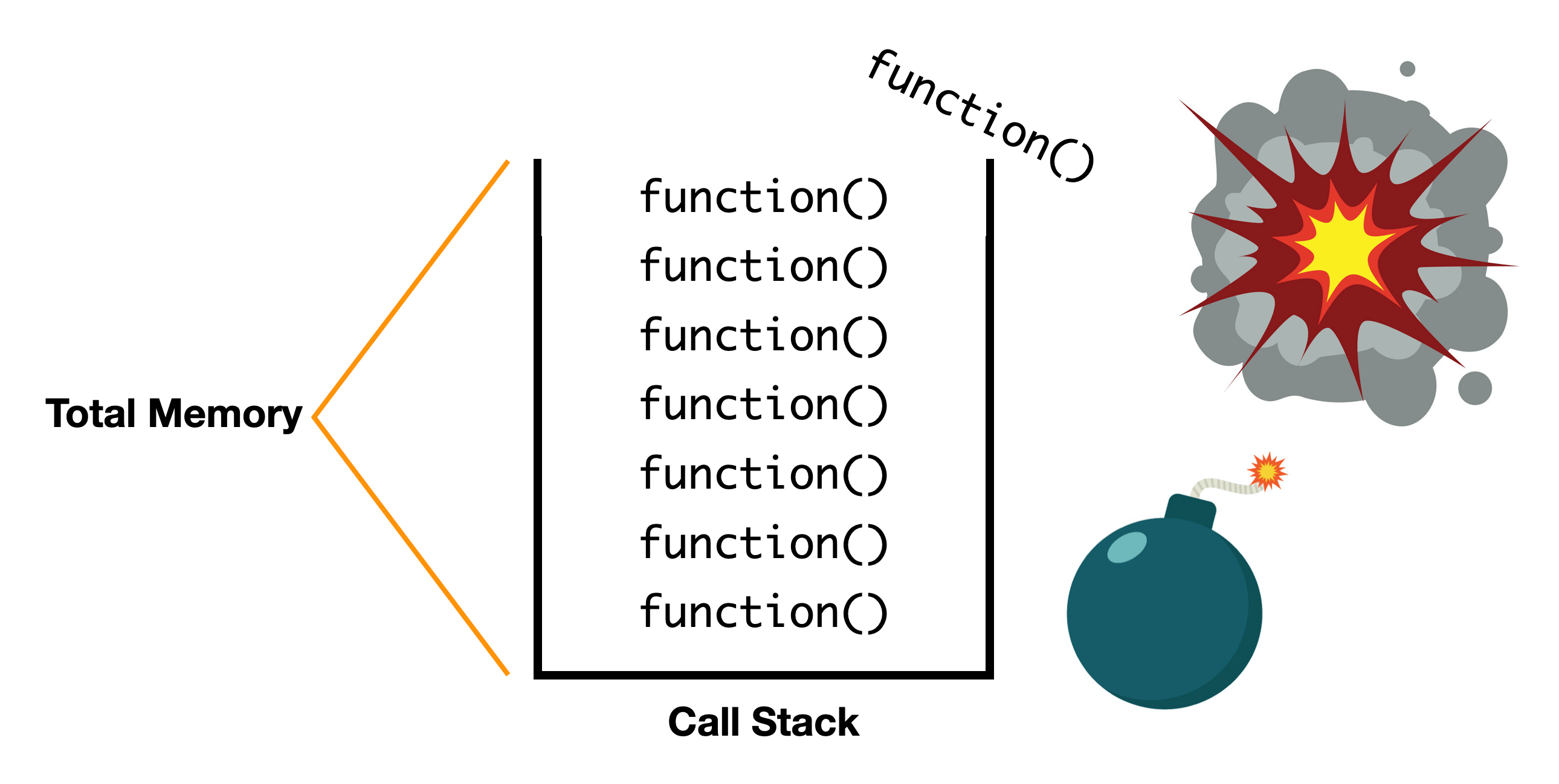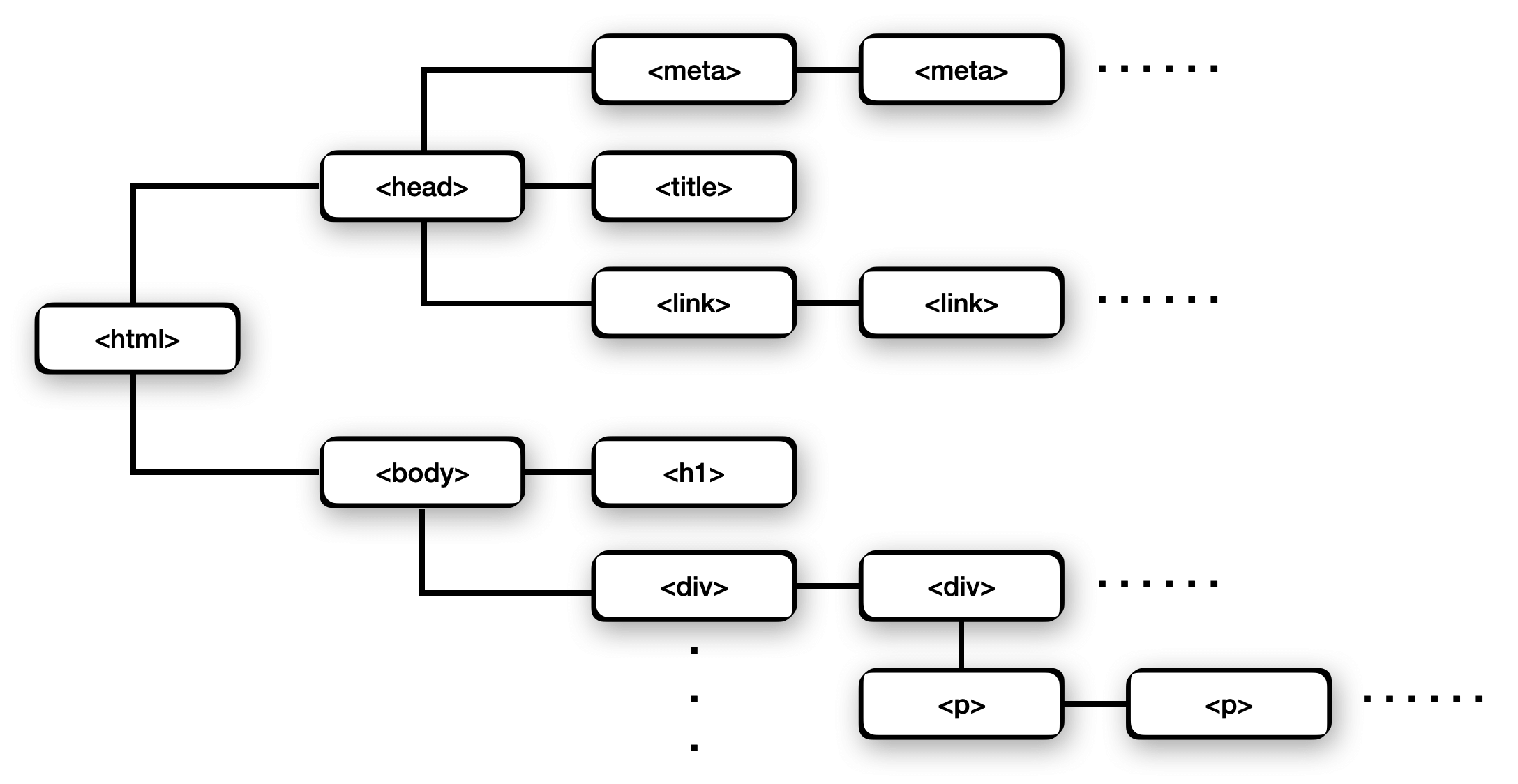Recursion | Skilled.dev
Learn

# Recursion

Recursion is when a function calls itself.

"In order to understand recursion, you must first understand recursion" - Anonymous

`` ``

As you work through this module, you will learn:

• How the iterative and recursive approach relate to each other.
• How recursion works.
• How to determine a stopping condition.
• Recognize when to use recursion.
• How to simplify complex problems using recursion.
• The tradeoffs and downside for using recursion.

You have probably heard of recursion, but it may not be obvious why it is important, how to think through a recursive function, or when to use it for a solution.

When we solve problems in interviews, we often repeat an operation many times, and once completed, this calculates the solution. This is our algorithm. For example, you have used the below patterns in your code:

`` ``

There are two strategies to implement our algorithms: iterative and recursive. The iterative approach is likely the one you are most familiar with. For example, looping through an array is a repeated operation, and we use the iterative `for` loop to perform this task for each item. Another iterative example is the `while` loop where we continue to repeat some modification until we reach a valid condition.

Recursion does the exact same thing as iteration, but it just takes a different approach. Anything that can be done iteratively can also be done recursively and vice versa.

For example, to calculate the factorial of a number iteratively vs. recursively:

`` ``

Let's visualize the recursive case. We start by calling `factorial(4)`. We recursively call the function with a smaller number each time until we reach the base case. Once hitting the base case, we always return `1`. So we return a constant value instead of continuing to call functions. Using this value, we can calculate the solution at each step and pass this result to the recursive function that called it until we reach our initial function call. When the initial execution gets the result from the recursive function it called, it can then calculate the final answer.Both the iterative and recursive functions here operate in O(n) time where `n` is the value of the integer.

With the factorial example, it likely still doesn't make sense why we need recursion. The iterative method seems more straightforward. Using recursion in this example may not add much value in terms of improving the solution, but there are many other cases where a recursive algorithm can reduce a very complex problem down to simple, repeatable steps that can solve a difficult question in just a few lines of code.

We will develop the intuition in this module and throughout the course on how/when to apply recursion and understand how it can simplify code.

## Coding a Recursive Function

There are two essential parts to the algorithms we use to solve interview questions:

1. Logic: the repeated operations that will determine the solution
2. Stopping condition: the condition that indicates we have found the solution

For recursion, we refer to these as the recursive case and the base case, if you are more familiar with those terms.

Let's expand on the factorial example since we already have some intuition for it.

If we take our iterative factorial algorithm, our logic was just doing `product = product * i`. The stopping condition was the `for` loop `i = 1; i <= factorial; i++`. We iterate until we have utilized every number required to calculate the factorial. If we wrote a malformed stopping condition, our `for` loop could run forever. For example, `let i = 1; i > 0; i++` will run infinitely because `i` will always be larger than 0.

Our recursive solution requires the exact same 2 parts. A stopping condition is essential to have in any recursive function. Just like in a `for` or `while` loop where we determine when our logic should stop executing, a recursive function must do the exact same thing.

In a recursive function, the "logic" and "stopping condition" can also be called the "recursive case" and "base case" respectively. The recursive case is the block of code that modifies the input, calls the function recursively, and uses the result returned from recursive function calls to build up the solution. The base case is executed when our input reaches a value that indicates we have completed all the steps needed to calculate the solution. The base case returns a pre-defined value (instead of calling the function recursively), and then all the recursive calls above it can unwind and use the calculated result from each step after it.

In our recursive factorial example, the base case is `number <= 1` which means we have multiplied all the numbers together for the factorial, and we can return `1`. The recursive case is `number * recursiveFactorial(number - 1)`.

For recursive functions, there are almost always two `return` statements — one for the base case and one for the recursive case.

Let's break this down to understand what it means and how our recursive factorial function works this way.

A factorial means we multiply an integer `n` by all the integers that come before it `1 to n`. A factorial is represented by the syntax `n!` where `n * (n-1) * (n-2) * ... * 1`.

Taking `1!` through `4!` as an example:

``````1! = 1
2! = 1 * 2
3! = 1 * 2 * 3
4! = 1 * 2 * 3 * 4
``````

We can immediately see that there is a lot of repetition here. `4!` is equivalent to `4 * 3!`, and `3! = 3 * 2!`. This holds true for every number until you reach `1!` which is just equivalent to itself.This is exactly why recursion works here. It is a bunch of subproblems that are steps to a larger problem, but if we solve them all individually and combine results, it calculates the answer.

To calculate `4!` we need `3!`. To calculate `3!` we need `2!`. To calculate `2!`, we need `1!`, which is just `1` and therefore the stopping condition.

This is exactly how we did it in our code:

`` ``

The difficult part about understanding recursion is that you need to think of the problem backward and that each execution is its own step. Each time you call the function, it needs the result of the function call after it, so it must wait until that result is calculated.

Since each step depends on a function call after it, these function calls stack up, and we must wait until it reaches the stopping condition which means it finally stops executing recursively. Then it passes the value from the stopping condition to the function call before, and this step can calculate its value. Then it yields the result to the step before it, and we unwind all the function calls this way until we reach our initial execution (`recursiveFactorial(4)`) which will now be able to calculate the result.

Being able to reduce a complex problem to a simple set of repeatable steps is when recursion becomes very powerful. Let's take a look in the next section at a case when recursion simplifies our solution.

## Practical Example of Recursion

To see when recursive is helpful, we will flatten an array. This is a very common scenario when you have nested items. For example, it is analogous to comments/replies in a thread or files/folders/subfolders on your computer.

We will also see that you can combine recursive and iterative techniques to build an optimal solution, as we do in the `recursiveFlatten` function.

At this point, you don't need to fully grasp what's happening in the functions. The important thing is to recognize that there are scenarios where recursion can simplify complex problems, and iteration can amplify them.

Given a dynamic array of integers or nested arrays of integers, write a function `flatten` that creates a one-dimensional array of integers.

`` ``

The iterative flatten algorithm is pretty complex. There is this `while` loop that will continue to run while we try to flatten the input. It creates a new data structure where we have to continually add and remove items determined by if the current item is an array or an integer.

On the other hand, our recursive solution just determines if each item is an array or not. If it is an array, it calls `recursiveFlatten` with that item and combines it with the output. Each level of nesting is handled as its own subproblem in the recursive solution, but the iterative solution must track the entire array while the algorithm flattens it.

The iterative approach works well when the solution is very linear and performs a set number of operations. However, the iterative approach starts to get complex when we need to iterate an unknown number of times or if there can be branching or nesting. Recursion performs very well in these cases because it just treats each iteration/nesting/branching as a new subproblem and solves it individually.

## Call Stack and Stack Overflow

We saw that with recursion, when we call functions in a nested manner, they must wait until the stopping condition is reached because each step needs the result from the function that comes after it.

This concept is known as the call stack, where each function call is added and creates a linear pile on top of each other. Since each consecutive function must wait on the result of another function before it can calculate the result for its own step, we're storing it in memory.

This means recursive solutions require O(n) space, where `n` is the size of the call stack — the number of recursive function calls for an arbitrarily large input.

We often don't know the number of recursive calls required before we start executing a recursive function, and since computers have limited memory, it's possible that the number of function calls added to the call stack grows larger than what the computer's memory can hold. Exceeding the size of memory available to the stack for function calls is known as a stack overflow and causes your program crash.We can see this happen by running the `doRecursion` function in the browser console.Stack overflows are a huge downside to recursive solutions, and this is something that is always important to mention in an interview. With the iterative solution, we don't build up a call stack in the same manner, so it operates with O(1) space as long as another data structure is not introduced.

If an iterative vs. recursive solution have similar readability in terms of code quality, it is preferred to use the iterative solution since stack overflows can be an issue. If a recursive solution is clearly more readable, or there is evidence to show the call stack won't overflow, you can (and may be expected to) use the recursive solution.

### Tail-Call Optimization

Some languages implement tail-call optimization where the recursive calls don't build a stack in memory, which reduces the space to O(1). This removes a downside of recursion of incurring space complexity and potentially causing a stack overflow.

The decision to include tail-call optimization is up to the language itself and often even the implementation of the language (ie. some versions of Python include it while others do not).

## Recursion in Coding Interviews

Recursion is seen in interviews in three forms:

1. Repeated tasks that can be viewed as smaller subproblems
2. Iterating a data structure that has unknown length, nesting depth, or branching
3. Searching and sorting algorithms

Dynamic programming is commonly associated with solving a complex problem by treating it as the combination of simple subproblems. You can use recursion with dynamic programming where you use memoization to store the result of recursive calls to prevent duplicate work. We have a dynamic programming module in this course where you will learn this concept thoroughly.

Traversing through data structures like linked lists, graphs, trees, and heaps is often done recursively. We often don't know the length, depth of nesting, or amount of branching, and recursion allows us to handle it simply by treating each node as its own step.

There is also an advanced technique known as backtracking that considers a tree of all possible results, recursively searches through them, and produces an optimal solution by considering all the possible outcomes.

Many searching and sorting methods break the input into smaller segments which directly aligns with how recursion approaches problems. You will see examples of this throughout the course.

Another term used to describe recursion is "divide and conquer". We break our problem into smaller subproblems, solve each locally, and combine them into a final answer.

Keep in mind potential tradeoffs and downside to recursion:

1. Possibility for stack overflow
2. Repeated operations which can degrade time complexity

Stack overflows may be unavoidable, but the repeated operations can be fixed with memoization.

Phrases that could indicate recursion is a candidate to solve a problem:

1. "Compute the `nth` value". Example: Calculate the `nth` Fibonacci number
2. "Calculate all the values". Example: Calculate all the permutations of a string
3. "Find a path between two points". Example: Given two points, determine the shortest path between them.

## Popular Recursion Examples

To gain a better intuition for recursion, this section lists some popular recursive functions.

Note that these functions don't implement optimizations like memoization that will be covered later in the course.

Reverse a string

`` ``

Calculate the nth Fibonacci number

`` ``

Tower of Hanoi

`` ``

## Recursion in the Real-World

Recursion is a fundamental topic in computer science, but it appears in nature as well. Examples of this include trees, snowflakes, and shells. They are the same pattern repeating horizontally and vertically to create their form. Many of these phenomena follow the Fibonacci sequence as well which is also a common recursive algorithm.Programming has very similar patterns. For example, the folder structure on your computer and the DOM tree on a website are both represented and iterated recursively. At any level there can be an infinite number of siblings or children.To walk the DOM purely iteratively, it would be incredibly challenging because we would have to track all the sibling and children nodes ourselves. However, using recursion we treat each level as a new subproblem, and if we encounter a child node, we recursively walk that. This simplifies iterating the DOM into just a few lines of basic code. Copy the function below in your browser terminal to see.

``````function recursivelyWalkDom(node) {
let currentNode = node;

if (currentNode.ELEMENT_NODE === 1) {
currentNode = currentNode.firstChild;

console.log(node.tagName);

while (currentNode) {
recursivelyWalkDom(currentNode);
currentNode = currentNode.nextSibling;
}
}
}

recursivelyWalkDom(document);
``````